NetworkX

#### Previous topic

average_clustering

Communities

# square_clustering¶

square_clustering(G, nodes=None)[source]

Compute the squares clustering coefficient for nodes.

For each node return the fraction of possible squares that exist at the node [R186]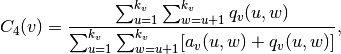where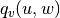are the number of common neighbors of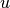and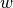other than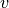(ie squares), and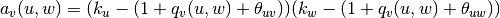, where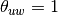ifandare connected and 0 otherwise.

Parameters : G : graph nodes : container of nodes, optional (default=all nodes in G) Compute clustering for nodes in this container. c4 : dictionary A dictionary keyed by node with the square clustering coefficient value.

Notes

While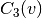(triangle clustering) gives the probability that two neighbors of node v are connected with each other,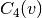is the probability that two neighbors of node v share a common neighbor different from v. This algorithm can be applied to both bipartite and unipartite networks.

References

 [R186] (1, 2) Pedro G. Lind, Marta C. González, and Hans J. Herrmann. 2005 Cycles and clustering in bipartite networks. Physical Review E (72) 056127.

Examples

>>> G=nx.complete_graph(5)
>>> print(nx.square_clustering(G,0))
1.0
>>> print(nx.square_clustering(G))
{0: 1.0, 1: 1.0, 2: 1.0, 3: 1.0, 4: 1.0}